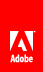# Convolution filter

Flash Player 9 and later, Adobe AIR 1.0 and later

 The ConvolutionFilter class can be used to apply a wide range of imaging transformations to BitmapData objects or display objects, such as blurring, edge detection, sharpening, embossing, and beveling. The convolution filter conceptually goes through each pixel in the source image one by one and determines the final color of that pixel using the value of the pixel and its surrounding pixels. A matrix, specified as an array of numeric values, indicates to what degree the value of each particular neighboring pixel affects the final resulting value. Consider the most commonly used type of matrix, which is a three by three matrix. The matrix includes nine values: ```N N N N P N N N N``` When the convolution filter is applied to a certain pixel, it will look at the color value of the pixel itself (“P” in the example), as well as the values of the surrounding pixels (labeled “N” in the example). However, by setting values in the matrix, you specify how much priority certain pixels have in affecting the resulting image. For example, the following matrix, applied using a convolution filter, will leave an image exactly as it was: ```0 0 0 0 1 0 0 0 0``` The reason the image is unchanged is because the original pixel’s value has a relative strength of 1 in determining the final pixel color, while the surrounding pixels’ values have relative strength of 0—meaning their colors don’t affect the final image. Similarly, this matrix will cause the pixels of an image to shift one pixel to the left: ```0 0 0 0 0 1 0 0 0``` Notice that in this case, the pixel itself has no effect on the final value of the pixel displayed in that location on the final image—only the value of the pixel to the right is used to determine the pixel’s resulting value. In ActionScript, you create the matrix as a combination of an Array instance containing the values and two properties specifying the number of rows and columns in the matrix. The following example loads an image and, when the image finishes loading, applies a convolution filter to the image using the matrix in the previous listing: ```// Load an image onto the Stage. var loader:Loader = new Loader(); var url:URLRequest = new URLRequest("http://www.helpexamples.com/flash/images/image1.jpg"); loader.load(url); this.addChild(loader); function applyFilter(event:MouseEvent):void { // Create the convolution matrix. var matrix:Array = [0, 0, 0, 0, 0, 1, 0, 0, 0]; var convolution:ConvolutionFilter = new ConvolutionFilter(); convolution.matrixX = 3; convolution.matrixY = 3; convolution.matrix = matrix; convolution.divisor = 1; loader.filters = [convolution]; } loader.addEventListener(MouseEvent.CLICK, applyFilter);``` Something that isn’t obvious in this code is the effect of using values other than 1 or 0 in the matrix. For example, the same matrix, with the number 8 instead of 1 in the right-hand position, performs the same action (shifting the pixels to the left). In addition, it affects the colors of the image, making them 8 times brighter. This is because the final pixel color values are calculated by multiplying the matrix values by the original pixel colors, adding the values together, and dividing by the value of the filter’s divisor property. Notice that in the example code, the divisor property is set to 1. As a general rule, if you want the brightness of the colors to stay about the same as in the original image, you should make the divisor equal to the sum of the matrix values. So with a matrix where the values add up to 8, and a divisor of 1, the resulting image is going to be roughly 8 times brighter than the original image. Although the effect of this matrix isn’t very noticeable, other matrix values can be used to create various effects. Here are several standard sets of matrix values for different effects using a three by three matrix: Basic blur (divisor 5): ``` 0 1 0 1 1 1 0 1 0``` Sharpening (divisor 1): ``` 0, -1, 0 -1, 5, -1 0, -1, 0``` Edge detection (divisor 1): ``` 0, -1, 0 -1, 4, -1 0, -1, 0``` Embossing effect (divisor 1): ``` -2, -1, 0 -1, 1, 1 0, 1, 2``` Notice that with most of these effects, the divisor is 1. This is because the negative matrix values added to the positive matrix values result in 1 (or 0 in the case of edge detection, but the divisor property’s value cannot be 0).Twitter™ and Facebook posts are not covered under the terms of Creative Commons.// Ethnio survey code removed